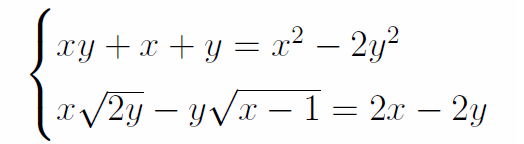# Another system of equations!

Algebra Level 1Find $x$ and $y$ with $x \geq 1$ and $y \geq 0$.

×